# RD Sharma Class 8 Solutions Chapter 14 Compound Interest Ex 14.3

In this chapter, we provide RD Sharma Class 8 Solutions Chapter 14 Compound Interest Ex 14.3 for English medium students, Which will very helpful for every student in their exams. Students can download the latest RD Sharma Class 8 Solutions Chapter 14 Compound Interest Ex 14.3 Maths pdf, free RD Sharma Class 8 Solutions Chapter 14 Compound Interest Ex 14.3 book pdf download. Now you will get step by step solution to each question.

### RD Sharma Solutions for Class 8 Chapter 14 Compound Interest Ex 14.3Download PDF

Question 1.
On what sum will the compound interest at 5% p.a. annum for 2 years compounded annually be Rs 164 ?
Solution:
Let Principal (P) = Rs 100
Rate (R) = 5% p.a.
Period (n) = 2 yearsQuestion 2.
Find the principal of the interest compounded annually at the rate of 10% for two years is Rs 210.
Solution:
Let principal (P) = Rs 100
Rate (R) = 10% p.a.
Period (n) = 2 yearsQuestion 3.
A sum amounts to Rs 756.25 at 10% per annum in 2 years, compounded annually. Find the sum.
Solution:
Amount (A) = Rs 756.25
Rate (R) = 10% p.a.
Period (n) = 2 yearsQuestion 4.
What sum will amount to Rs 4913 in 18 months, if the rate of interest is 1212 % per annum, compounded half-yearly.
Solution:
Amount (A) = Rs 4,913Question 5.
The difference between the compound interest and simple interest on a certain sum at 15% per annum for 3 years is Rs 283.50. Find the sum.
Solution:
Let sum (P) = Rs 100
Rate (R) = 15% p.a.
Period (n) = 3 yearsQuestion 6.
Rachna borrowed a certain sum at the rate of 15% per annum. If she paid at the end of two years Rs 1,290 as interest compounded annually, find the sum she borrowed.
Solution:
C.I. = Rs 1,290
Rate (R) = 15% p.a.
Period (n) = 2 years
Let sum (P) = Rs 100Question 7.
The interest on a sum of Rs 2,000 is being compounded annually at the rate of 4% per annum. Find the period for which the compound interest is Rs 163.20.
Solution:
Sum (P) = Rs 2,000
C.I. = Rs 163.20
Amount (A) = P + C.I. = Rs 2000 + Rs 163.20 = Rs 2163.20
Rate (R) = 4%
Let period = n years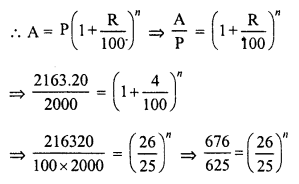Question 8.
In how much time would Rs 5,000 amount to Rs 6,655 at 10% per annum compound interest ?
Solution:
Principal (P) = Rs 5,000
Amount (A) = Rs 6,655
Rate (R) = 10%
Let period = n years.Question 9.
In what time will Rs 4,400 becomes Rs 4,576 at 8% per annum interest compounded half-yearly ?
Solution:
Principal (P) = Rs 4,400
Amount (A) = Rs 4,576
Rate (R) = 8% or 4% half-yearly
Let period = n half-yearsn = 1
Period = 1 half year

Question 10.
The difference between the S.I. and C.I. on a certain sum of money for 2 years at 4% per annum is Rs 20. Find the sum.
Solution:
Difference between C.I. and S.I. = Rs 20
Rate (R) = 4% p.a.
Period (n) = 2 years
Let principal (P) = Rs 100Question 11.
In what time will Rs 1,000 amount to Rs 1,331 at 10% per annum compound interest.
Solution:
Principal (P) = Rs 1,000
Amount (A) = Rs 1,331
Rate (R) = 10% p.a.
Let period = n yearQuestion 12.
At what rate percent compound interest per annum will Rs 640 amount to Rs 774.40 in 2 years ?
Solution:
Principal (P) = Rs 640
Amount (A) = Rs 774.40
Period (n) = 2 years.
Let R be the rate of interest p.a.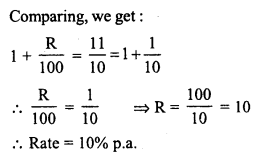Question 13.
Find the rate percent per annum if Rs 2000 amount to Rs 2,662 in 112 years, interest being compounded half-yearly ?
Solution:
Principal (P) = Rs 2,000
Amount (A) = Rs 2,662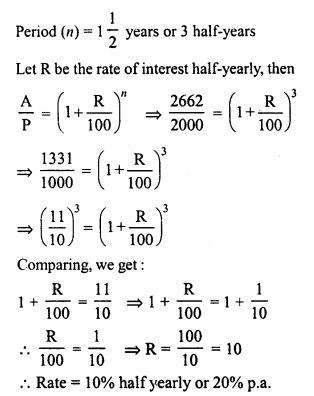Question 14.
Kamala borrowed from Ratan a certain rate for two years simple interest. She lent this sum at the same rate to Hari for two years compound interest. At the end of two years, she received Rs 210 as compound interest, but paid Rs 200 only as simple interest. Find the sum and the rate of interest.
Solution:
Simple interest = Rs 200
and compound interest = Rs 210.
Period = 2 yearsQuestion 15.
Find the rate percent per annum, if Rs 2,000 amount to Rs 2,315.25, in an year and a half, interest being compounded six monthly.
Solution:
Principal (P) = Rs 2,000
Amount (A) = Rs 2315.25Question 16.
Find the rate at which a sum of money will double itself in 3 years, if the interest is compounded annually.
Solution:
Let Principal (P) = Rs 100
then Amount (A) = Rs 200
Period (n) = 3 years
Let R be the rate % p.a.Question 17.
Find the rate at which a sum of money will become four times the original amount in 2 years, if the interest is compounded half- yearly.
Solution:
Let Principal (P) = Rs 100
Then Amount (A) = Rs 400
Period (n) = 2 years or 4 half years
Let R be the rate % half-yearly, thenRate % = 41.42% half yearly and 82.84% p.a.

Question 18.
A certain sum amounts to Rs 5,832 in 2 years at 8% compounded interest. Find the sum.
Solution:
Amount (A) = Rs 5,832
Let P be the sum
Rate (R) = 8% p.a.
Period (n) = 2 yearsQuestion 19.
The difference between the compound interest and simple interest on a certain sum for 2 years at 7.5% per annum is Rs 360. Find the sum.
Solution:
Let sum (P) = Rs 100Question 20.
The difference in simple interest and compound interest on a certain sum of money at 623 % per annum for 3 years is Rs 46. Determine the sum.
Solution:
Let sum (P) = Rs 100Question 21.
Ishita invested a sum of Rs 12,000 at 5% per annum compound interest. She received an amount of Rs 13,230 after n years, Find the value of n.
Solution:
Principal (P) = Rs 12,000
Amount (A) = Rs 13,230
Rate (R) = 5% p.a.
Period = n yearsQuestion 22.
At what rate percent per annum will a sum of Rs 4,000 yield compound interest of Rs 410 in 2 years ?
Solution:
Principal (P) = Rs 4,060
C.I. = Rs 410
Amount (A) = Rs 4,000 + 410 = Rs 4,410
Let rate = R % p.a.
Period (n) = 2 years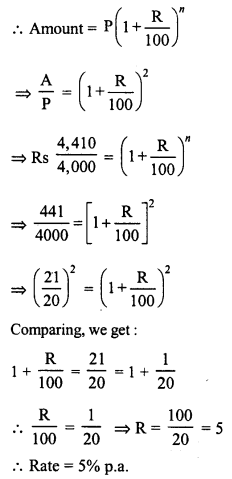Question 23.
A sum of money deposited at 2% per annum compounded annually becomes Rs 10404 at the end of 2 years. Find the sum deposited.
Solution:
Amount (A) = Rs 10,404
Rate (R) = 2% p.a.
Period (n) = 2 years.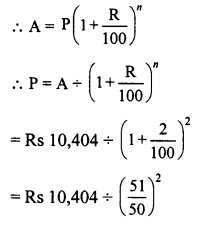Question 24.
In how much time will a sum of Rs 1,600 amount to Rs 1852.20 at 5% per annum compound interest ?
Solution:
Principal (P) = Rs 1,600
Amount (A) = Rs 1852.20
Rate (R) = 5% p.a.
Let n be the timeQuestion 25.
At what rate percent will a sum of Rs 1,000 amount to Rs 1102.50 in 2 years at compound interest ?
Solution:
Principal (P) = Rs 1,000
Amount (A) = Rs 1102.50 .
Period (n) = 2 years
Let R be the rate of interest p.a.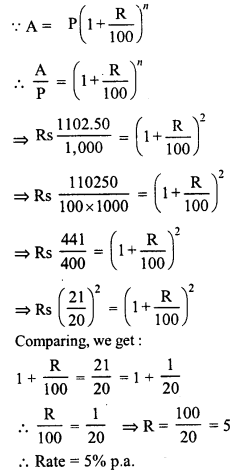Question 26.
The compound interest on Rs 1,800 at 10% per annum for a certain period of time is Rs 378. Find the time in years.
Solution:
Principal (P) = Rs 1,800
C.I. = Rs 378
Amount (A) = P + C.I. = Rs 1,800 + 378 = Rs 2,178
Rate (R) = 10% p.a.
Let n be the period in yearsComparing, we get:
n = 2
Period = 2 years

Question 27.
What sum of money will amount to Rs 45582.25 at 634 % per annum in two years, interest being compounded annually ?
Solution:
Amount (A) = Rs 45582.25Question 28.
Sum of money amounts to Rs 4,53,690 in 2 years at 6.5% per annum compounded annually. Find the sum.
Solution:
Amount (A) = Rs 4,53,690All Chapter RD Sharma Solutions For Class 8 Maths

—————————————————————————–

All Subject NCERT Exemplar Problems Solutions For Class 8

All Subject NCERT Solutions For Class 8

*************************************************

I think you got complete solutions for this chapter. If You have any queries regarding this chapter, please comment on the below section our subject teacher will answer you. We tried our best to give complete solutions so you got good marks in your exam.

If these solutions have helped you, you can also share rdsharmasolutions.in to your friends.